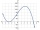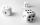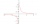# Two cubes

The surfaces of two cubes, one of which has an edge of 22 cm longer than the second are differ by 19272 cm2. Calculate the edge length of both cubes.

Result

a =  84 cm
b =  62 cm

#### Solution:

$a = 22+b \ \\ 6 \cdot \ a^2 - 6 \cdot \ b^2 = 19272 \ \\ \ \\ 6 \cdot \ a^2 - 6 \cdot \ (a-22)^2 = 19272 \ \\ 264a = 22176 \ \\ a = 22176 / 264 = 84 \ \\ \ \\ a = 84 = 84 \ \text { cm }$
$b = a-22 = 84-22 = 62 = 62 \ \text { cm }$Our examples were largely sent or created by pupils and students themselves. Therefore, we would be pleased if you could send us any errors you found, spelling mistakes, or rephasing the example. Thank you!

Leave us a comment of this math problem and its solution (i.e. if it is still somewhat unclear...):Be the first to comment!#### Following knowledge from mathematics are needed to solve this word math problem:

Looking for help with calculating roots of a quadratic equation? Do you have a linear equation or system of equations and looking for its solution? Or do you have quadratic equation?

## Next similar math problems:

1. Boxes200 boxes have been straightened in three rows. The first was 13 more than in the second, and in the second was one fifth more than in the third one. How many boxes are in each row?
2. Ball gameRichard, Denis and Denise together scored 932 goals. Denis scored 4 goals over Denise but Denis scored 24 goals less than Richard. Determine the number of goals for each player.
3. Reciprocal valueHow do I calculate a number x that is 9 greater than its reciprocal (1/x)?
4. Three diceWhen you throw three dice was the sum total of the dice 10. The yellow dice fell one eye more than on the red and brown fell 3 eyes less than red. How many eyes fell on every dice?
5. EquationEquation ? has one root x1 = 8. Determine the coefficient b and the second root x2.
6. GP - three membersThe second and third of a geometric progression are 24 and 12(c+1) respectively, given that the sum of the first three terms of progression is 76 determine value of c
7. Equation 23Find value of unknown x in equation: x+3/x+1=5 (problem finding x)
8. Linsys2Solve two equations with two unknowns: 400x+120y=147.2 350x+200y=144Find the roots of the quadratic equation: 3x2-4x + (-4) = 0.
10. RootsDetermine the quadratic equation absolute coefficient q, that the equation has a real double root and the root x calculate: ?
11. Linear systemSolve this linear system (two linear equations with two unknowns): x+y =36 19x+22y=720
12. Square root 2If the square root of 3m2 +22 and -x = 0, and x=7, what is m?
13. Football match 4In a football match with the Italy lost 3 goals with Germans. Totally fell 5 goals in the match. Determine the number of goals of Italy and Germany.
14. DiscriminantDetermine the discriminant of the equation: ?
15. Evaluation of expressionsIf a2-3a+1=0, find (i)a2+1/a2 (ii) a3+1/a3
16. Solve 3Solve quadratic equation: (6n+1) (4n-1) = 3n2
17. Theorem proveWe want to prove the sentence: If the natural number n is divisible by six, then n is divisible by three. From what assumption we started?